## Main

In the case of a homogeneous Bose–Einstein condensate (BEC) with pure contact interaction, the existence of a stable ground state depends on the modulus and sign of the interaction. For repulsive interaction (a>0), the BEC is stable, whereas for attractive interaction (a<0), the BEC is unstable. This instability can be prevented by an external trapping potential. The tendency of the gas to shrink towards the centre of the trap is in that case counteracted by the repulsive quantum pressure arising from the Heisenberg uncertainty relation. Detailed analysis8 shows that in a harmonic trap with mean frequency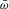, a condensate is stable as long as the number of atoms N stays below a critical value Ncrit given by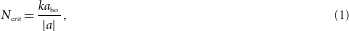where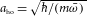is the harmonic oscillator length and k is a constant of the order of 1/2. This scaling has been experimentally checked in ref. 9 in a BEC of 85Rb. The dynamics of condensates for NNcrit has been the subject of several experiments with condensates of 7Li (refs 10, 11) and 85Rb (ref. 12). In refs 13, 14, the collapse of a Bose–Fermi mixture of 87Rb and 40K was investigated. Some aspects of the dynamics such as the soliton train formation in 7Li (ref. 15) and 85Rb (ref. 16) remain the subject of ongoing research.

Being anisotropic and long range, the dipole–dipole interaction (DDI) differs significantly from the contact interaction, which changes the stability conditions in a system with DDI present. Considering a purely dipolar condensate with homogeneous density polarized by an external field, it is found that owing to the anisotropy of the DDI, the BEC is unstable, independent of how small the dipole moment is17. As in the pure contact case, a trap helps to stabilize the system. In the dipolar case, however, it is not only the quantum pressure that prevents the collapse but also the anisotropy of the density distribution imprinted by the trap.

Consider a cylindrically symmetric harmonic trap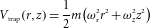with the dipoles oriented along z, and r being the distance from the symmetry axis. As can be intuitively understood from Fig. 1a, in a pancake-shaped trap (aspect ratio λ=ωz/ωr>1), the dipoles predominantly repel each other and the BEC is stable. In contrast, a cigar-shaped trap (λ<1, Fig. 1b) leads to mainly attractive forces and hence to a dipolar collapse. Following this simple argument, we expect that in the prolate case a positive scattering length a is needed to stabilize the BEC, whereas in the oblate case, we can even afford a slightly negative a. The dependence of the stability of a dipolar BEC on the trap aspect ratio λ and scattering length a has been extensively studied theoretically4,5,6,7, and is experimentally investigated here.Figure 1: Intuitive picture of the trap geometry dependence of the stability of a dipolar BEC.

Our measurements are carried out with a BEC of 52Cr (ref. 18), which is so far the only experimentally accessible quantum gas with observable DDI19,20. To compare contact and dipolar interactions, we introduce a length scale characterizing the magnetic DDI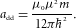The numerical prefactor in add is chosen such that a homogeneous condensate becomes unstable to local density perturbations for aadd (ref. 21). As chromium has a magnetic dipole moment of μ=6μB (μB is the Bohr magneton), add15a0, where a0 is the Bohr radius. Far from Feshbach resonances, a takes its background value abg100a0 (ref. 22) and the BEC is stable for any λ. To explore the unstable regime, we thus reduce the scattering length a, which in the vicinity of a Feshbach resonance scales as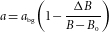with the applied magnetic field B.

To carry out the measurements, we produce a BEC of approximately 25,000 atoms at a magnetic field that is about 10 G above the broadest Feshbach resonance where the scattering length is approximately 85a0 and the BEC is stable. Once the BEC is obtained20,23, we adiabatically shape the trapping potential to the desired aspect ratio λ. To be able to vary λ over a wide range, we generate the trapping potential by a crossed optical dipole trap (ODT) and a superimposed one-dimensional optical lattice along the z direction (see Fig. 1c and Methods section).

We observe two effects when approaching the zero-crossing of the scattering length: the BEC shrinks in both directions owing to the decreasing scattering length and the ellipticity of the cloud changes as a manifestation of the enhanced dipolar effects20. Finally, when we decrease the scattering length below some critical value acrit, the BEC atom number (determined from a bimodal fit24 of the time-of-flight absorption images) abruptly decreases (Fig. 2a,b). The disappearance of the BEC around the instability point is shown in Fig. 2c. Although slightly above acrit, we still see an almost pure BEC, for aacrit the density shows a bimodal distribution (an anisotropic, dense central peak surrounded by an isotropic gaussian cloud). Just below acrit, the BEC collapses and the density distribution becomes a unimodal, isotropic gaussian. Note that we do not observe the formation of soliton trains as in refs 15, 16. This can be attributed to the fact that as our trap is much tighter than in those references, the initial size of our BEC is smaller than any single soliton observed in refs 15, 16.Figure 2: Decrease of the BEC atom number N around the critical scattering length acrit.

The critical scattering length acrit where the condensate vanishes depends strongly on the trap aspect ratio λ. For an isotropic trap (Fig. 2a), the collapse occurs at a15a0, whereas the pancake-shaped trap (Fig. 2b) can even stabilize a purely dipolar BEC (a0). We repeated this experiment for six different traps (see Table 1), thereby covering a range of two orders of magnitude in the trap aspect ratio λ. To exclude three-body loss processes causing the abrupt decrease in the BEC atom number, we measured the lifetime of the BEC for the different traps just above acrit and found the same lifetime (10 ms) for the different scattering lengths.

By fitting to the observed BEC atom numbers (Fig. 2a,b) the threshold function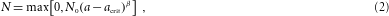where N0, acrit and β are fitting parameters, we find the critical scattering length acrit. The simple functional form (2) was empirically chosen as it accounts for the fast decreasing BEC atom number at aacrit and for the slow decrease for aacrit. The exponent β describing the steepness of the collapse was found to be β0.2 for all traps. The values of acrit that are plotted in Fig. 3a mark the experimentally obtained stability threshold of a 52Cr BEC in the plane (λ,a). We observe a clear shift towards smaller a as λ increases. For the most oblate trap (λ=10), we can reduce the scattering length to zero and hence access the purely dipolar regime experimentally.Figure 3: Stability diagram of a dipolar BEC in the a–λ plane.

To get a more quantitative insight into the collapse threshold acrit(λ), we numerically determine the critical scattering length (green curve in Fig. 3a). For this, we use a variational method to minimize the Gross–Pitaevskii energy functional1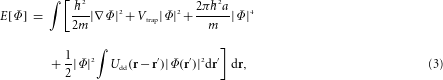where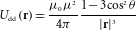is the interaction energy of two aligned magnetic dipoles μ, with r being the relative position of the dipoles and θ the angle between r and the direction z of polarization.

Similar to the work presented in refs 4, 5, 6 and 25, we use a cylindrically symmetric gaussian ansatz to evaluate the energy functional (3) with the radial and axial widths σr and σz as variational parameters (see the Methods section). To obtain acrit, we lower the scattering length until the energy landscape E(σr,σz) does not contain any minimum for finite σr and σz any more (Fig. 3b–e). Starting with large values a>add, we find that E(σr,σz) supports a global minimum for finite σr and σz independently of λ and thus the BEC is stable (Fig. 3b). Going below aadd, the absolute ground state is a collapsed infinitely thin cigar-shaped BEC (σr→0) and the possible existence of a further local minimum (corresponding to a metastable state) is determined by the trap aspect ratio λ (see Fig. 3c, where add>a>acrit and Fig. 3d, where a=acrit). Finally, below a−2add (Fig. 3e), the local minimum vanishes for any λ and the BEC is always unstable6,7.

In spite of the simplicity of our model, we find good agreement between experiment and theory (Fig. 3a). We checked that the different atom numbers and mean trap frequencies that we find for the six traps modify the green curve by much less than the error bars.

The behaviour of the critical scattering length acrit as a function of the aspect ratio λ can be understood considering the limit N add/aho1, which is satisfied by our average experimental value of N add/aho23. Owing to their linear N-scaling, the kinetic energy and the potential energy (equations (5) and (6) in the Methods section) can be neglected and the total energy E(σr,σz) is dominated by the interaction term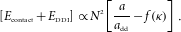The function f (ref. 25) of the cloud aspect ratio κ=σr/σz arises from the DDI and is discussed in the Methods section.

In this regime, where the stability is solely governed by the competition between the contact and DDI, the critical scattering length (grey curve in Fig. 3a) is implicitly given by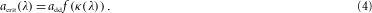The asymptotic behaviour of the theory curve now becomes apparent: an extremely prolate (oblate) trap forces the cloud shape to also be extremely prolate (oblate) and f takes its extremal value 1 (−2). Hence, we find the asymptotic values acrit=add for λ→0 and acrit=−2add for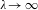. Another particular point is acrit=0, marking the aspect ratio λc needed to stabilize a purely dipolar BEC. More precisely, as f(1)=0, we search for the trap in which the ground state of a purely dipolar BEC is isotropic. As the DDI tends to elongate the BEC along the z direction and shrink it radially26, it is clear that the desired trap is oblate. Using our model we obtain the criterion λ>λc≈5.2 for a purely dipolar BEC to be stable, a result that agrees well with the values found in refs 2, 4, 5, 6, 7.

The grey curve in Fig. 3a that we obtain by numerically solving equation (4) shows a universal behaviour in the sense that in the large-N limit acrit(λ) does not depend anymore on the absolute values of the trap frequencies and N. This fact clearly distinguishes the dipolar collapse from the pure contact case (red curve in Fig. 3a), where the λ-dependence, which is already weak for finite N (ref. 27), completely vanishes in the limit of large N as the stability criterion reads acrit(λ)=0 (see equation (1) and red curve in Fig. 3a). Furthermore, the instability threshold obtained here applies for any dipolar system in a harmonic potential, such as, for example, heteronuclear molecules, where the only difference is the specific value of add.

In summary, we experimentally mapped the stability diagram of a dipolar BEC. The dependence on scattering length and trap aspect ratio agrees well with a simple model based on the minimization of the energy of a gaussian ansatz. By using a pancake-shaped trap, we were able to enter the regime of purely dipolar quantum gases. Although the lifetime of the purely dipolar BEC is relatively short (10 ms), this work opens up the route to new and exciting physics2. A clear subject for future studies is the dynamics of the dipolar collapse, which might show anisotropic features. Another remarkable property predicted to appear in a dipolar BEC contained in a pancake-shaped trap is the existence of a roton minimum in its Bogoliubov spectrum21. Furthermore, close to the collapse threshold, the existence of structured ground states is predicted28,29, a precursor for the supersolid phase30 that is expected to appear in dipolar BECs in three-dimensional optical lattices. Finally, a field that has gained increasing interest in the recent past is the study of unusual vortex lattice patterns in rotating dipolar BECs31,32.

## Methods

### Experimental set-up and procedure

To be able to vary λ over a wide range, the trapping potential is generated by a crossed ODT20,23 and a further optical lattice (Fig. 1c). The two lattice beams (wavelength λlatt=1,064 nm, waist wlatt=110 μm, maximum power per beam Platt=5 W) propagate in the xz plane under a small angle of ϑ/2=4 with respect to the x axis. This configuration creates a standing wave along the z axis with a spacing d=λlatt/[2sin(ϑ/2)]=7.6 μm. Owing to the large spacing of the lattice and the small size of the BEC (Thomas–Fermi diameter 2RzTF6 μm), we load at most two sites when ramping up the optical lattice. The resulting change in the atom number (at most dividing by 2) changes the theoretical value of acrit by less than a0, which is below the size of our experimental error bars. Tunnelling processes are completely negligible on the timescale of our experiments.

By varying the powers in the beams, we are able to provide nearly cylindrically symmetric traps, with aspect ratios λ between 1/10 and 10, while keeping the average trap frequency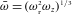approximately constant. More extreme aspect ratios are not used as for extreme oblate (prolate) traps the radial (axial) confinement becomes too weak to hold the atoms against gravity and remaining magnetic field gradients. The properties of the six traps that were used in the experiment are listed in Table 1. The cylindrical symmetry of the oblate traps in the xy direction is given by the fact that ωx and ωy are mainly determined by the horizontal ODT beam, whereas ωz is given by the lattice and the vertical beam is not used. For prolate traps, ωx and ωy are again mainly given by the horizontal ODT beam, whereas the vertical beam determines ωz and the lattice is not used. For all traps, except trap 3, we find 0.94<ωx/ωy<1.04. For trap 3, the critical scattering length acrit has been calculated using a gaussian ansatz with the three widths σx,y,z as variational parameters. The obtained value deviates by less than a0 from the value found with the cylindrically symmetric ansatz.

After the BEC is obtained by forced evaporation in the ODT, the trapping potential is shaped in 25 ms to the desired aspect ratio λ. We then ramp the magnetic field within 10 ms to adjust the value of the scattering length. To tune a accurately, we use the broadest of the resonances in 52Cr (ref. 22), which is located at B0589 G and has a width of ΔB1.5 G (ref. 20). The current providing the magnetic field is actively stabilized with a remaining noise of less than 1×10−5 r.m.s., which results in a resolution of Δaa0 around the zero crossing of the scattering length. After a further holding time of 2 ms, we finally switch off the trap and take an absorption image along the x axis, after a time-of-flight of 5 ms. The BEC atom number N and radii Ry,z are obtained by fitting the density profile using a bimodal distribution23. The calibration between scattering length and magnetic field is done by solving the hydrodynamic equations for the scattering length a with known N and Ry,z (refs 20, 26).

### Calculation of the critical scattering length

To obtain an estimate of acrit, we calculate the energy E(σr,σz) (3) of the cylindrically symmetric gaussian wave function4,5,6,25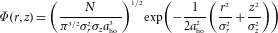with σr and σz as variational parameters. Using this ansatz, where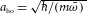, the contributions to the total energy are5,6 the zero-point fluctuations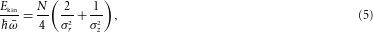the potential energy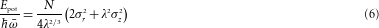and the mean-field interaction energy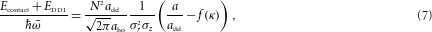with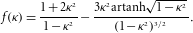The function f(κ) is a monotonically decreasing function of the condensate aspect ratio κ=σr/σz with the asymptotic values f(0)=1 and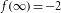, arising from the non-local term in equation (3) (ref. 25). It vanishes for κ=1, implying that for an isotropic density distribution the magnetic DDI does not contribute to the total energy. As the interaction term (7) scales as N2, it overrules the other terms in the limit N add/aho1.